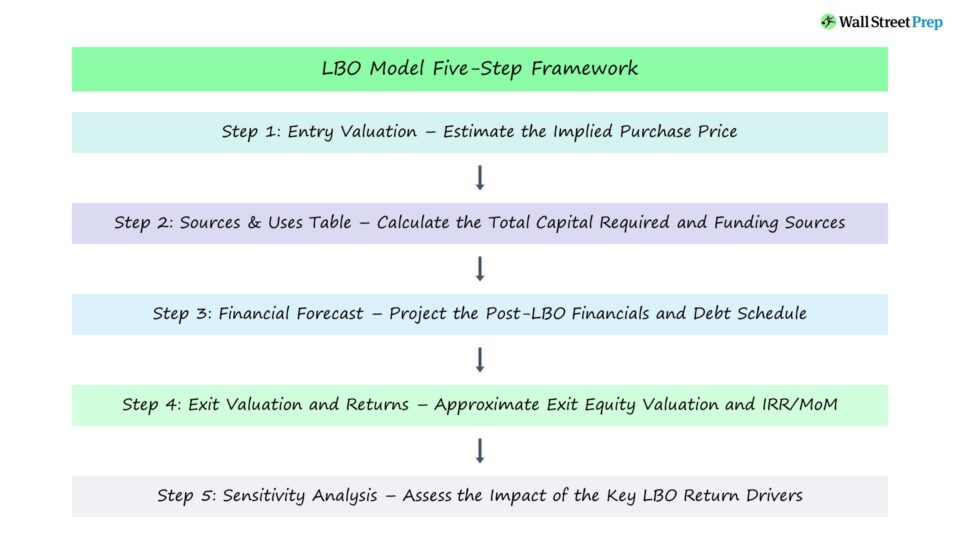Welcome to Wall Street Prep! Use code at checkout for 15% off.# Walk Me Through an LBO Model

Guide to Understanding the Steps to Building an LBO Model## How to Build an LBO Model?

An LBO model estimates the implied returns from the buyout of a target company by a financial sponsor – i.e. private equity firm – in which a significant portion of the purchase price is funded with debt capital.

Following the buyout, the firm operates the post-LBO company for around five to seven years – with the free cash flows (FCFs) of the company used to pay down more debt each year.

The following information should be determined from an LBO model:

## Step 1. LBO Entry Valuation

Imagine you’re currently in the process of recruiting for the buy-side and the interviewer sitting across from you asked the following question:

• “Walk me through an LBO model?”

So, the first step to build an LBO model is to calculate the implied entry valuation based on an entry multiple assumption.

To calculate the enterprise value at entry, the entry multiple is multiplied by either the last twelve months (LTM) EBITDA of the target company or the next twelve months (NTM) EBITDA.

• Entry Valuation = Purchase EBITDA x Entry Multiple

If we assume a “cash-free, debt-free” transaction, then the calculated enterprise value is the purchase price of the LBO target.

## Step 2. Sources and Uses Schedule

All else being equal, the lower the required upfront equity contribution from the financial sponsor, the higher the returns.

The next step is to create the sources & uses schedule, which approximates:

• “Uses” Side: The total amount of capital required to complete the acquisition
• “Sources” Side: The specific details on how the firm plans to come up with the required funding

The majority of the “uses” side will be attributable to the buyout of the target’s existing equity. But in addition, other transaction assumptions are made, such as:

From here, numerous financing assumptions are made regarding the sources of funds, such as the:

• Total Debt Financing (i.e. Leverage Multiple, Senior Leverage Multiple)
• Lending Terms for Each Debt Tranche (e.g. Interest Rate Pricing, Required Amortization, Cash Sweep)
• Management Rollover Assumptions
• Cash to B/S (i.e. Excess Cash)

The remaining amount for the sources & uses side to be equal is the equity contributed by the financial sponsor (i.e. the “plug”).

## Step 3. Financial Forecast and Debt Schedule

In the subsequent step, the financial performance of the company is projected for a minimum five-year time horizon, which is the standard holding period assumed for modeling purposes.

A complete 3-statement model is required for the LBO assumptions to properly impact the income statement and cash flow statement (i.e. the free cash flow build).

The debt schedule is used to closely track the following:

For the LBO model to calculate the returns accurately, the debt schedule must adjust each debt tranche accordingly to determine the amount of debt paid down in each period (and the ending balances).

## Step 4. LBO Exit Valuation and Returns Schedule (IRR and MOIC)

Next, the assumptions regarding the exit must be made – most notably, the exit EV/EBITDA multiple.

In practice, the conservative assumption is to set the exit multiple equal to the purchase multiple.

Upon calculating the exit enterprise value using the exit multiple assumption and exit year EBITDA, the remaining net debt on the balance sheet as of the presumed date of exit can be deducted to arrive at the exit equity value.

After calculating the exit equity value attributable to the sponsor, the key LBO return metrics – i.e. the internal rate of return (IRR) and multiple of money (MoM) – can be estimated.

## Step 5. LBO Sensitivity Analysis

In the final step, different operating cases must be considered – e.g. a “Base Case”, “Upside Case”, and a “Downside Case” – along with sensitivity analyses to assess how adjusting certain assumptions impacts the implied returns from the LBO model.

The entry multiple and exit multiples are usually the two assumptions with the most impact on returns, followed by the leverage multiple and other operational characteristics (e.g. revenue growth, margins).

Master LBO Modeling Our Advanced LBO Modeling course will teach you how to build a comprehensive LBO model and give you the confidence to ace the finance interview.